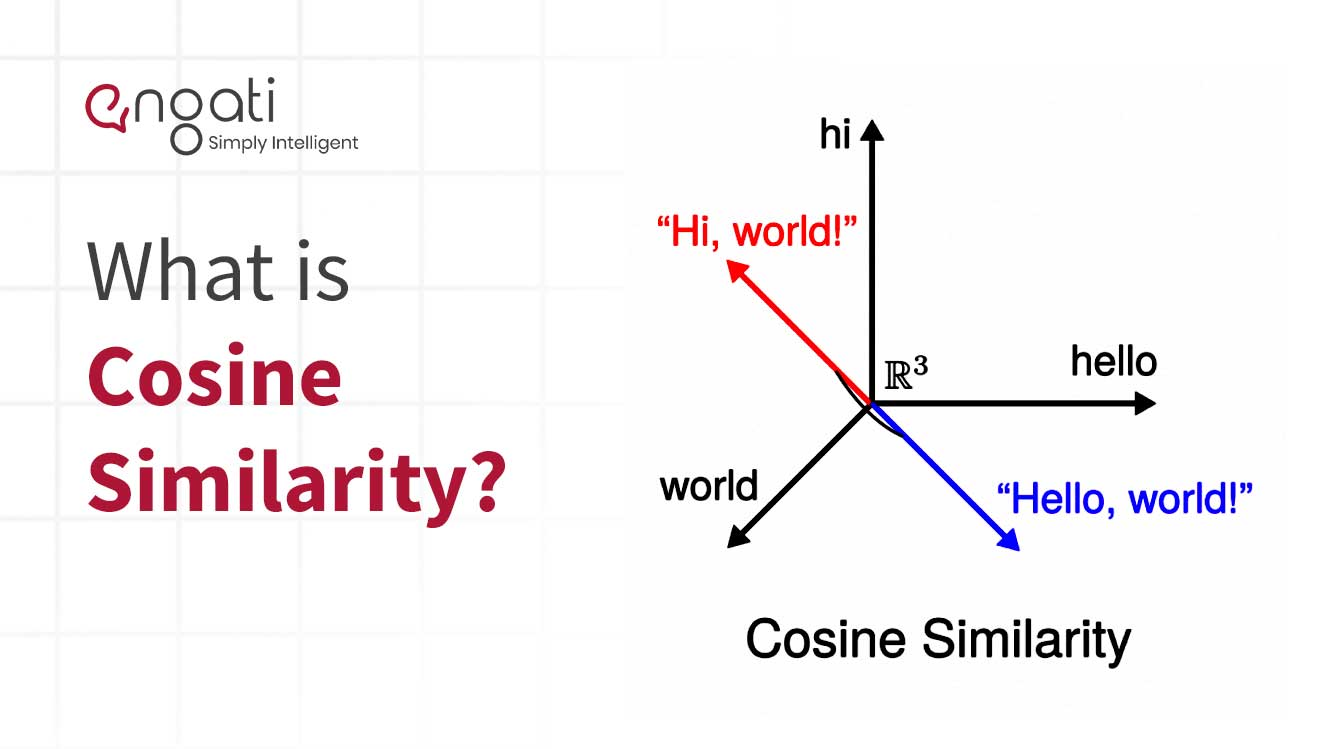# Cosine similarity## What is cosine similarity?

Cosine similarity measures the similarity between two vectors of an inner product space. It is measured by the cosine of the angle between two vectors and determines whether two vectors are pointing in roughly the same direction. It is often used to measure document similarity in text analysis.

The mathematical equation of Cosine similarity between two non-zero vectors is:

## How does cosine similarity work?

Cosine Similarity is a value that is bound by a constrained range of 0 and 1.

The similarity measurement measures the cosine of the angle between the two non-zero vectors A and B.

Suppose the angle between the two vectors was 90 degrees. In that case, the cosine similarity will have a value of 0; this means that the two vectors are orthogonal or perpendicular to each other.

As the cosine similarity measurement gets closer to 1, the angle between the two vectors, A and B, is smaller. The images below depict this more clearly.

## Why do we use cosine similarity in NLP?

In NLP, Cosine similarity is a metric used to measure how similar the documents are irrespective of their size. Mathematically, it calculates the cosine of the angle between two vectors projected in a multi-dimensional space.

The cosine similarity is advantageous because even if the two similar documents are far apart by the Euclidean distance because of the size (for example, the word “chatbot” could appear 50 times in one document and 10 times in another), they could still have a smaller angle between them. Smaller the angle, the higher the similarity.

## How is cosine similarity used?

### 1. Document Similarity

A scenario that involves identifying the similarity between pairs of a document is a good use case for the utilization of cosine similarity as a quantification of the measurement of similarity between two objects.

Quantification of the similarity between two documents can be obtained by converting the words or phrases into a vectorized form of representation.

The vector representations of the documents can then be used within the cosine similarity formula to obtain a quantification of similarity.

In the scenario described above, the cosine similarity of 1 implies that the two documents are exactly alike. A cosine similarity of 0 would conclude that there are no similarities between the two documents.

### 2. Pose Matching

Pose matching involves comparing the poses containing critical points of joint locations.

Pose estimation is a computer vision task, and it’s typically solved using Deep Learning approaches such as Convolutional Pose Machine, Stacked hourglass, PoseNet, etc.Request a Demo!
Get started on Engati with the help of a personalised demo.
This is some text inside of a div block.
This is some text inside of a div block.
This is some text inside of a div block.
This is some text inside of a div block.
*only for sharing demo link on WhatsApp
Thanks for the information.
We will be shortly getting in touch with you.
Oops! something went wrong!
For any query reach out to us on contact@engati.comI am looking for a conversational AI engagement solution for the web and other channels.I would like for a conversational AI engagement solution for WhatsApp as the primary channelI am an e-commerce store with Shopify. I am looking for a conversational AI engagement solution for my businessI am looking to partner with Engati to build conversational AI solutions for other businessesYou're a step away from building your Al chatbot

How many customers do you expect to engage in a month?

Less Than 2000

2000-5000

More than 5000Thanks for the information.

We will be shortly getting in touch with you.